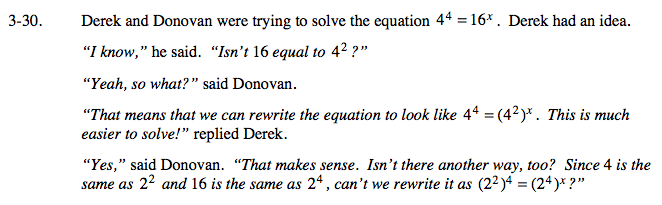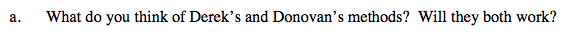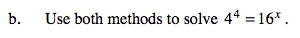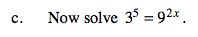### Home > CAAC > Chapter 14 > Lesson 14.3.1.2 > Problem3-30

3-30.
1. Derek and Donovan were trying to solve the equation 44 = 16x . Derek had an idea.
“I know,” he said. “Isn't 16 equal to 42 ?”
“Yeah, so what?” said Donovan.
“That means that we can rewrite the equation to look like 44 = (42)x. This is much easier to solve!” replied Derek.
“Yes,” said Donovan. “That makes sense. Isn't there another way, too? Since 4 is the same as 22 and 16 is the same as 24 ,
can't we rewrite it as
(22)4 = (24)x?” Homework Help ✎

1. What do you think of Derek's and Donovan's methods? Will they both work?

2. Use both methods to solve 44 = 16 x .

3. Now solve 35 = 92x .Are these methods violating any rules or properties of exponents?x = 2x = 1.25